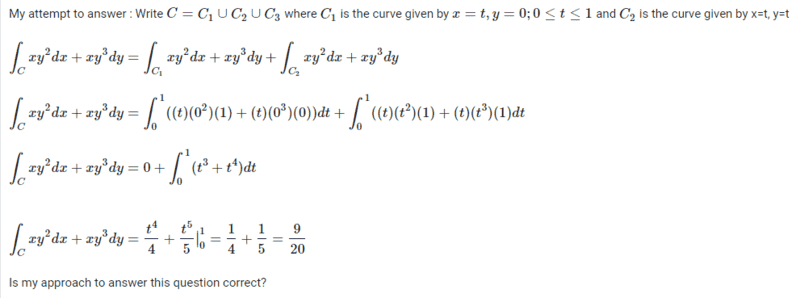# Computing##\displaystyle\int_C f\cdot dr ## for the given vector field

• WMDhamnekar

#### WMDhamnekar

MHB
Homework Statement
##f(x,y) =xy^2i + xy^3 j ; ## C: the ploygonal path from (0,0) to (1,0) to (0,1) to (0,0)
Relevant Equations
NonePlease refrain from posting your work as images. Even if legible (which it hardly is on my phone without zooming in) it is impossible to quote properly. Instead use the built-in LaTeX functions and type your work.

That said, you have not specified ##C_3## and your ##C_2## does not correspond to any part of the desired curve.

•WMDhamnekar
The integral for ##C_3## should be zero, still you should 've said that explicitly.

I think your parameterization ##x=t,y=t## for ##C_2## is wrong (according to that parameterization the point (1,1) for t=1 belongs to the path, but we know it does NOT), I think the correct one is ##x=t,y=1-t##.

•WMDhamnekar
I think the correct one is x=t,y=1−t.
This is not correct. Signs matter. OP should be able to deduce the correct path.

This is not correct. Signs matter. OP should be able to deduce the correct path.
Sorry I don't see a sign error, its the straight line path from (1,0) to (0,1) (t has to vary from 1 to 0 though not from 0 to 1).

•WMDhamnekar
Sorry I don't see a sign error, its the straight line path from (1,0) to (0,1) (t has to vary from 1 to 0 though not from 0 to 1).
OP has all integrals from 0 to 1. Unless explicitly stated that would be the typical assumption.

•Delta2
Write ##C = C_1 \cup C_2 \cup C_3## where C1 is the curve given by x = t, y =0, 0≤t≤1 , C2 is the curve given by x= (1-t), y =t, 0≤ t ≤1, and C3 is the curve given by x=0, y = 1-t, 0≤ t≤ 1. Then,

## \displaystyle\int_C xy^2 dx +xy^3 dy= \displaystyle\int_{C_1} xy^2 dx + xy^3 dy + \displaystyle\int_{C_2}xy^2 dx +xy^3 dy + \displaystyle\int_{C_3}xy^2 dx + xy^3 dy ##

## \displaystyle\int_C xy^2 dx +xy^3 dy = \displaystyle\int_0^1 t(0^2)(1) + t(0^3)(0) dt + \displaystyle\int_0^1(1-t)(t^2)(-1) + (1-t)(t^3)(1) dt + \displaystyle\int_0^1 0 (0^2) 0 + 0(0^3)0dt##

## \displaystyle\int_C xy^2 dx +xy^3 dy = 0 +\displaystyle\int_0^1 -t^2 +2t^3 -t^4 dt +0##

## \displaystyle\int_C xy^2 dx +xy^3 dy = -\frac{t^3}{3} + 2\frac{t^4}{4} - \frac{t^5}{5} \big|_0^1##
## \displaystyle\int_C xy^2 dx +xy^3 dy = -\frac13 +\frac12 -\frac15 =-\frac{1}{30}##

If correct, why is the answer negative? What does this negative answer indicate?

Yes it seems correct to me
You should be able to infer what the negative sign means. When does the dot product ##\mathbf{f}\cdot\mathbf{dr}## becomes negative?

Yes.
If correct, why is the answer negative? What does this negative answer indicate?
That you went backwards, in a sense.

•WMDhamnekar
PS In general ##\int_a^b = -\int_b^a##. And, if the integrand is positive and ##a < b## then ##\int_a^b## is positive.

@PeroK your interpretation though correct is a bit shallow , there is something a bit more deep relating on how the vector field's direction relates to the direction we walk the path.

@PeroK your interpretation though correct is a bit shallow , there is something a bit more deep relating on how the vector field's direction relates to the direction we transverse the path.
In general, it won't be easy to see whether the answer is positive or negative. But, if you have a positive integrand in the first quadrant, then left to right and upwards is positive; right to left and upwards is negative (what we have here); left to right and downwards is negative; and, right to left and downwards is positive.

•WMDhamnekar
In general, it won't be easy to see whether the answer is positive or negative. But, if you have a positive integrand in the first quadrant, then left to right and upwards is positive; right to left and upwards is negative (what we have here); left to right and downwards is negative; and, right to left and downwards is positive.
I admit you confused me a bit here, but you seem to be thinking in terms of the integral we get after we do the parameterization.

I would say to think in terms of the line integral representing the work of a force.

•WMDhamnekar
It is true that a work integral takes the form of a line integral, but the concept is of course much more general than that. Mathematically, the circulation integral being negative can be roughly interpreted as the field being integrated pointing more against the curve direction than in the curve direction on average. The circulation integral being non-zero also let's us conclude that the field is not conservative (and hence cannot be written as the gradient of a scalar field etc).

•Delta2 and PeroK